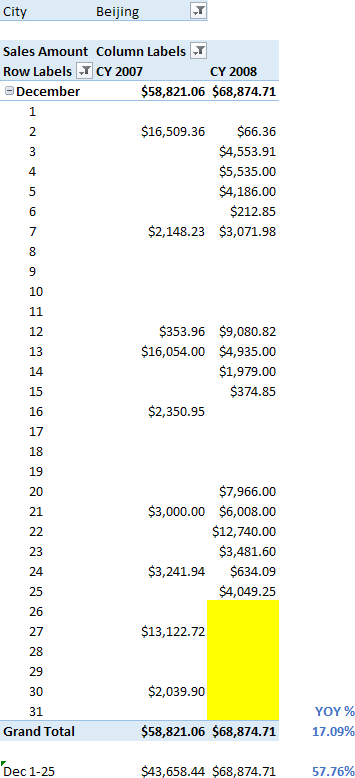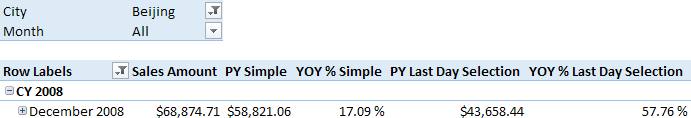When you create a year-over-year in DAX, you usually compare two set of dates from the calendar, regardless of the presence of data in all the days of the period. For example, consider the following year-over-year (YOY) calculation for Sales in December 2008 for a particular store.The total for December shows the sum of all the days. However, if you do not have data after December 25, 2008, you might want to compare only the same range of days (December 1 to 25) in the year-over-year comparison. As you see in the picture, the comparison between equivalent periods would result in a 57.76% increase, whereas the comparison using all the days in the month has a lower growth (17.09%).

The calculation of the year-over-year percentage (YOY %) is based on the previous year (PY) measure, as in the following example using the standard time intelligence function SAMEPERIODLASTYEAR:

```[PY Simple] :=
CALCULATE (
[Sales Amount],
SAMEPERIODLASTYEAR ( 'Date'[Date] )
)

[YOY % Simple] :=
DIVIDE ( [Sales Amount] - [PY Simple], [PY Simple] )

```

If you want to consider only the days where both years have sales for the current selection (in this case, a single store), then you can write the following measures.

```[PY Last Day Selection] :=
VAR LastDaySelection =
LASTNONBLANK ( 'Date'[Date], [Sales Amount] )
VAR CurrentRange =
DATESBETWEEN ( 'Date'[Date], MIN ( 'Date'[Date] ), LastDaySelection )
VAR PreviousRange =
SAMEPERIODLASTYEAR ( CurrentRange )
RETURN
IF (
LastDaySelection >= MIN ( 'Date'[Date] ),
CALCULATE ( [Sales Amount], PreviousRange )
)

[YOY % Last Day Selection] :=
DIVIDE ( [Sales Amount] - [PY Last Day Selection], [PY Last Day Selection] )
```

If you are using Excel 2010/2013 or Analysis Services Tabular 2012/2014, you have to write the PY Last Day Selection without the variables. This result in a less efficient code.

```[PY Last Day Selection] :=
IF (
LASTNONBLANK ( 'Date'[Date], [Sales Amount] ) >= MIN ( 'Date'[Date] ),
CALCULATE (
[Sales Amount],
SAMEPERIODLASTYEAR (
DATESBETWEEN (
'Date'[Date],
MIN ( 'Date'[Date] ),
LASTNONBLANK ( 'Date'[Date], [Sales Amount] )
)
)
)
)
```

Such a calculation is very dynamic and it results in the desired comparison.However, another approach could be looking for the last day available for any store. You can obtain this by modifying the LASTNONBLANK filter, including all the stores, as in the following measures.

```[PY Last Day Absolute] :=
VAR LastDayAbsolute =
LASTNONBLANK (
'Date'[Date],
CALCULATE ( [Sales Amount], ALLEXCEPT ( Sales, 'Date' ) )
)
VAR CurrentRange =
DATESBETWEEN ( 'Date'[Date], MIN ( 'Date'[Date] ), LastDayAbsolute )
VAR PreviousRange =
SAMEPERIODLASTYEAR ( CurrentRange )
RETURN
IF (
LastDayAbsolute >= MIN ( 'Date'[Date] ),
CALCULATE ( [Sales Amount], PreviousRange )
)

[YOY % Last Day Absolute] :=
DIVIDE ( [Sales Amount] - [PY Last Day Absolute], [PY Last Day Absolute] )
```

Also in this case, if you are using Excel 2010/2013 or Analysis Services Tabular 2012/2014, you cannot use the variables.

```[PY Last Day Absolute] :=
IF (
LASTNONBLANK ( 'Date'[Date], [Sales Amount] ) > MIN ( 'Date'[Date] ),
CALCULATE (
[Sales Amount],
SAMEPERIODLASTYEAR (
DATESBETWEEN (
'Date'[Date],
MIN ( 'Date'[Date] ),
LASTNONBLANK (
'Date'[Date],
CALCULATE ( [Sales Amount], ALLEXCEPT ( Sales, 'Date' ) )
)
)
)
)
)
```

Sometimes, you can use a simplified version of the filter for PY Last Day Absolute measure, leveraging on the current day, or the previous day, for example using this approach for the variable CurrentRange:

```VAR CurrentRange =
DATESBETWEEN ( 'Date'[Date], MIN ( 'Date'[Date] ), TODAY () - 1 )
```

However, if for any reason you do not receive updated data, the dynamic measure extends the range of the comparison in the previous year even if the data available do not have the latest days. The approach shown in this article is data-driven and ignores the current calendar date, which might result more reliable if you might have delays in populating data for your model.

You can download the sample file below, which contains the versions in Excel 2013, Excel 2016, and Power BI.